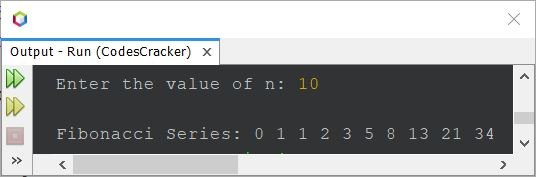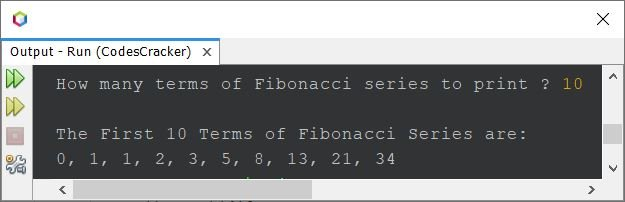# Java Program to Print Fibonacci Series

This article covers a program in Java that prints Fibonacci series. Fibonacci series are a series of numbers in which the first two terms are 0 and 1, and rest terms are formed in a way that the next term is the sum of previous two terms. For example, 0, 1, 1, 2, 3, 5, 8 and so on.

## Print Fibonacci Series in Java upto n Terms

The question is, write a Java program to print Fibonacci series upto n. The value of n must be received by user at run-time of the program. For example, if user enters 5 as value of n, then the program should print first 5 terms of Fibonacci series. The program given below is its answer:

```import java.util.Scanner;

public class CodesCracker
{
public static void main(String[] args)
{
int a=0, b=1, c=0, n;
Scanner s = new Scanner(System.in);

System.out.print("Enter the value of n: ");
n = s.nextInt();

System.out.print("\nFibonacci Series: " +a+ " " +b+ " ");
c = a+b;
n = n-2;
while(n>0)
{
System.out.print(c+ " ");
a = b;
b = c;
c = a+b;
n--;
}
}
}```

The snapshot given below shows the sample run of above Java program with user input 10 as value of n to find and print first 10 numbers/terms of Fibonacci series:#### Modified Version of Previous Program

The above program looks weird, as I've done some manual things like printing of first two terms of Fibonacci series manually. Also there is a limitation of above program. That is, what if user enters the value of n as 0 or 1 ?
then also the program prints first two terms of Fibonacci series. Therefore let's modify the above program and create a new one as shown below:

```import java.util.Scanner;

public class CodesCracker
{
public static void main(String[] args)
{
int a, b=1, c=0, n, i;
Scanner s = new Scanner(System.in);

System.out.print("How many terms of Fibonacci series to print ? ");
n = s.nextInt();

System.out.println("\nThe First " +n+ " Terms of Fibonacci Series are:");
for(i=1; i<=n; i++)
{
if(i==1)
c = 0;
else if(i==2 || i==3)
c = 1;
else
{
a = b;
b = c;
c = a+b;
}
if(i==n)
System.out.print(c);
else
System.out.print(c+ ", ");
}
}
}```

Here is its sample run with same user input as of previous program:## Print Fibonacci Series upto 100 using for Loop

This program prints Fibonacci series upto 100. That is, the last term of Fibonacci series must be less than or equal to 100. The program is created using for loop.

```import java.util.Scanner;

public class CodesCracker
{
public static void main(String[] args)
{
int a, b=1, c=0, i;
Scanner s = new Scanner(System.in);

for(i=1; c<=100; i++)
{
if(i==1)
c = 0;
else if(i==2 || i==3)
c = 1;
else
{
a = b;
b = c;
c = a+b;
}
if(c<=100)
System.out.print(c+ " ");
}
}
}```

The sample output of above Java program is:

`0 1 1 2 3 5 8 13 21 34 55 89 `

## Print Fibonacci Series upto 100 using while Loop

This is the same program as of previous. The only difference is, it is created using while loop, instead of for

```import java.util.Scanner;

public class CodesCracker
{
public static void main(String[] args)
{
int a, b=1, c=0, i=1;
Scanner s = new Scanner(System.in);

while(c<=100)
{
if(i==1)
c = 0;
else if(i==2 || i==3)
c = 1;
else
{
a = b;
b = c;
c = a+b;
}
if(c<=100)
System.out.print(c+ " ");
i++;
}
}
}```

#### Same Program in Other Languages

Java Online Test

« Previous Program Next Program »